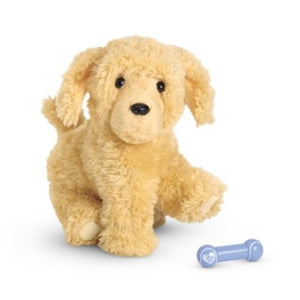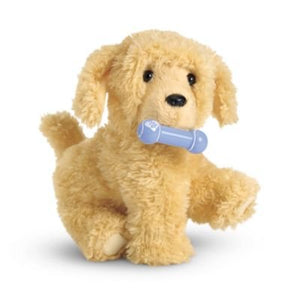# American Girl Pet - Apricot Poodle Puppy - Truly Me 2015

Vendor
American Girl
Regular price
\$98.55
Sale price
\$89.61
Quantity must be 1 or more

• E

• v

• e

• r

• y

• d

• o

• l

• l

• l

• o

• v

• e

• s

• a

• p

• l

• a

• y

• f

• u

• l

• p

• e

• t

• -

• a

• n

• d

• t

• h

• i

• s

• p

• r

• e

• t

• t

• y

• a

• p

• r

• i

• c

• o

• t

• p

• o

• o

• d

• l

• e

• p

• u

• p

• p

• y

• i

• s

• a

• f

• o

• r

• e

• v

• e

• r

• f

• r

• i

• e

• n

• d

• .

• S

• h

• e

• h

• a

• s

• s

• o

• f

• t

• f

• u

• r

• a

• n

• d

• a

• b

• o

• d

• y

• t

• h

• a

• t

• c

• a

• n

• b

• e

• p

• o

• s

• e

• d

• i

• n

• d

• i

• f

• f

• e

• r

• e

• n

• t

• p

• o

• s

• i

• t

• i

• o

• n

• s

• .

• A

• m

• a

• g

• n

• e

• t

• i

• n

• h

• e

• r

• m

• o

• u

• t

• h

• l

• e

• t

• s

• h

• e

• r

• h

• o

• l

• d

• a

• f

• a

• v

• o

• r

• i

• t

• e

• t

• o

• y

• .

• Color : peach

Every American Girl doll loves a playful pet-and this pretty apricot poodle puppy is a forever friend. She has soft fur and a body that can be posed in different positions. A magnet in her mouth lets her hold a favorite toy.